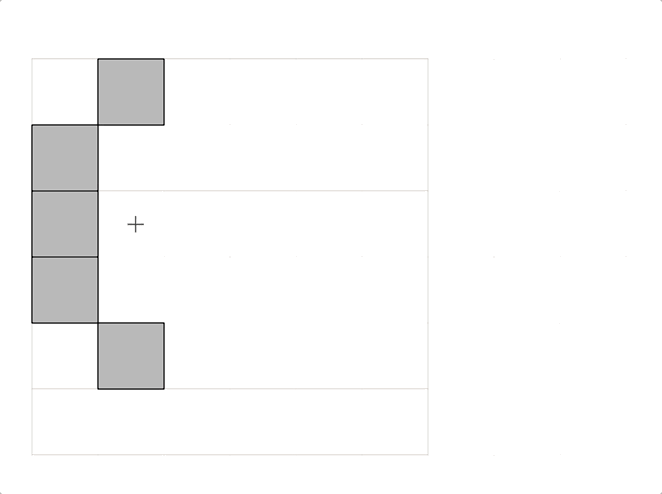# Pixel tool deletes wrong pixels

Edit: Georg’s Pixel toolglyphs 2 Version 2.6.5 (1342)
rota2.glyphs (10.0 KB)

Which pixel plugin do you have?

yours, Georgs

The pixels are rotated and flipped. That makes it one step more complicated.
You can run this script to normalise the components:

``````for c in Layer.components:
scale = c.scale
c.scale = (1, 1)
rotation = c.rotation
c.rotation = 0
p = c.position
if scale < 0:
p.x -= 100
if scale < 0:
p.y -= 100
if rotation == -90:
p.x -= 100
c.position = p
``````
2 Likes

thanks,
just one more question:
Can this be run only for the components named ‘pixel’?

``````for c in Layer.components:
if c.componentName != "pixel":
continue
scale = c.scale
c.scale = (1, 1)
rotation = c.rotation
c.rotation = 0
p = c.position
if scale < 0:
p.x -= 100
if scale < 0:
p.y -= 100
if rotation == -90:
p.x -= 100
c.position = p
``````
1 Like!

on run, they move 100pixels to the left, but it’s fine, thanks, I’ll leave them as is